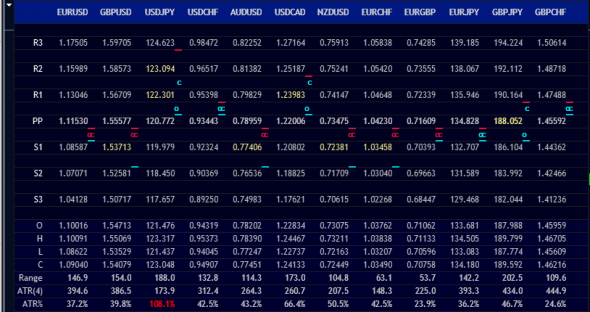# Free Downoad Multi Pair Pivot Point Levels and ATR Indicator For Mt4

This indicator will display simultaneously pivot point levels and the percentage of ATR has been achieved by currency pairs you choose. As you know that the pivot points can be used to identify levels of support and resistance. Coupled with reached ATR percentage can increase the likelihood of a change of price movements, breakout or continuation.

There are two types of pivot points that are used in this indicator, Standard and Fibonacci. Most traders know how its calculated, but I’ll show it anyway.

## Pivot Point Calculation

Standard Pivot Point:
R3 = H + 2(PP – L)
R2 = PP + (H – L)
R1 = (2 x PP) – L
PP = (H + L + C) / 3
S1 = (2 x PP) – H
S2 = PP – (H – L)
S3 = Low – 2(H – PP)

Fibonacci Pivot Point:
PP = (H + L + C) / 3
R3 = PP + ((H – L) x 1.000)
R2 = PP + ((H – L) x .618)
R1 = PP + ((H – L) x .382)
S1 = PP – ((H – L) x .382)
S2 = PP – ((H – L) x .618)
S3 = PP – ((H – L) x 1.000)

Abbr:
C – Closing Price
H – High
L – Low
PP – Pivot Point
S1 – First Support
S2 – Second Support
S3 – Third Support
R1 – First Resistance
R2 – Second Resistance
R3 – Third Resistance

## Indicator: Multi Pair Pivot Point Levels and ATR

Parameters:

• Symbol Sets, default value: EURUSD, GBPUSD, USDJPY, USDCHF, AUDUSD, USDCAD, NZDUSD, EURCHF, EURGBP, EURJPY, GBPJPY, GBPCHF. A list of pairs separated by comma.
• Timeframe, default value: Daily.
• Pivot Type, default value: Standard.
• ATR Period, default value: 5.
• Position – Top, default value: 0, top position of dashboard panel relative to chart frame container.
• Position – Left, default value: 12, left position of dashboard panel relative to chart frame container.

Let’s see what information we can get from the picture above. Visually we can see that the USDJPY on the weekly time frame has the following data:

• Opening price is between PP and R1
• The highest price this week is between R2 and R3
• Lowest price this week is between PP and R1
• Current price this week is between R1 and R2
• ATR 4 weekly has been reached.
Try to use symbol sets of EUR pairs (EURAUD, EURCAD, EURCHF, EURGBP, EURJPY, EURNZD, EURUSD). You can see the pair is being led or everything just like being locked up between R1 and S1, as shown below.